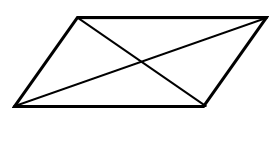QuestionAnswers

# Given four distinct points in a plane. How many maximum numbers of lines can be drawn through them?A) 6B) 7C) 10D) 4Verified
128.4k+ views
Hint: If there are n distinct points in a plane, then we take the help of combination to find the number of lines that can be drawn from them, by taking any two random points at a time we can find the number of lines possible.

Complete step by step solution: Given, 4 distinct points in a plane.
The number of combination, i.e., number of ways of selecting ’r’ elements out of ‘n’ is mathematically expressed as
${}^{\text{n}}{{\text{C}}_{\text{r}}}{\text{ = }}\dfrac{{{\text{n!}}}}{{{\text{r!}}\left( {{\text{n - r}}} \right){\text{!}}}}$
Now, we have given four distinct points.
Therefore, we have to find the number of ways of selecting any two points at random, mathematically i.e.,
${}^{\text{4}}{{\text{C}}_{\text{2}}}{\text{ = }}\dfrac{{{\text{4!}}}}{{{\text{[(4 - 2)!](2!)}}}} \\ {\text{ = }}\dfrac{{{\text{4!}}}}{{{\text{(2!)(2!)}}}} \\ {\text{ = }}\dfrac{{{\text{(4)(3)(2)(1)}}}}{{{\text{(2)(1)(2)(1)}}}} \\ {\text{ = (3)(2)}} \\ {\text{ = 6}} \\$

Therefore, (A) 6 is the correct answer.

${}^{\text{n}}{{\text{C}}_{\text{r}}}{\text{ = }}\dfrac{{{\text{n!}}}}{{{\text{r!}}\left( {{\text{n - r}}} \right){\text{!}}}}$From the above solution, it shows ${}^{\text{4}}{{\text{C}}_{\text{2}}}{\text{ = 6}}$ and we have 6 lines joining the 4 random points shown in the above figure.